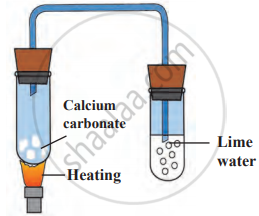# 20. Explain the reaction given in figure. - Science and Technology 1

Explain the reaction given in the figure.#### Solution

1. Calcium carbonate undergoes a decomposition reaction in the first test tube forming calcium oxide powder and carbon dioxide gas.
$\ce{\underset{\text{Limestone}}{CaCO_{3(s)}} ->[\Delta] \underset{\text{Calcium oxide}}{CaO_{(s)}} + \underset{\text{Carbon dioxide}}{CO2\uparrow}}$
2. Carbon dioxide gas formed in the first test tube turns the lime water milky in the second test tube due to the formation of calcium carbonate, which is insoluble in water.
$\ce{\underset{\text{Calcium hydroxide}}{Ca(OH)_{2(aq)}} + \underset{\text{Carbon dioxide}}{CO_{2(g)}} -> \underset{\text{Calcium carbonate}}{CaCO_{3(s)}} + \underset{\text{Water}}{H2O_{(l)}}}$
3. The calcium oxide powder, remains behind in the first test tube.
Concept: Types of Chemical Change or Chemical Reaction - Decomposition Reactions
Is there an error in this question or solution?